Chapter Notes: Pair of Linear Equations in two Variables

# Pair of Linear Equations in two Variables Chapter Notes - Mathematics (Maths) Class 10

 Table of contentsLinear EquationsPair of linear equations in two variablesGraphical Representation of Linear EquationSolving Pair of Linear EquationsSubstitution  MethodElimination MethodCross Elimination  Method (Deleted from NCERT)Solving Pair of Linear Equations - Graphical Method (Deleted from NCERT)Equations Reducible to Linear Equations in Two Variables (Deleted from NCERT)## Linear Equations

Linear equations can be used to represent almost any situation involving an unknown quantity.
We apply linear equations in various real-life situations like weather predictions, ingredients of a recipe, our monthly expenditure, predicting profit in business and Government surveys.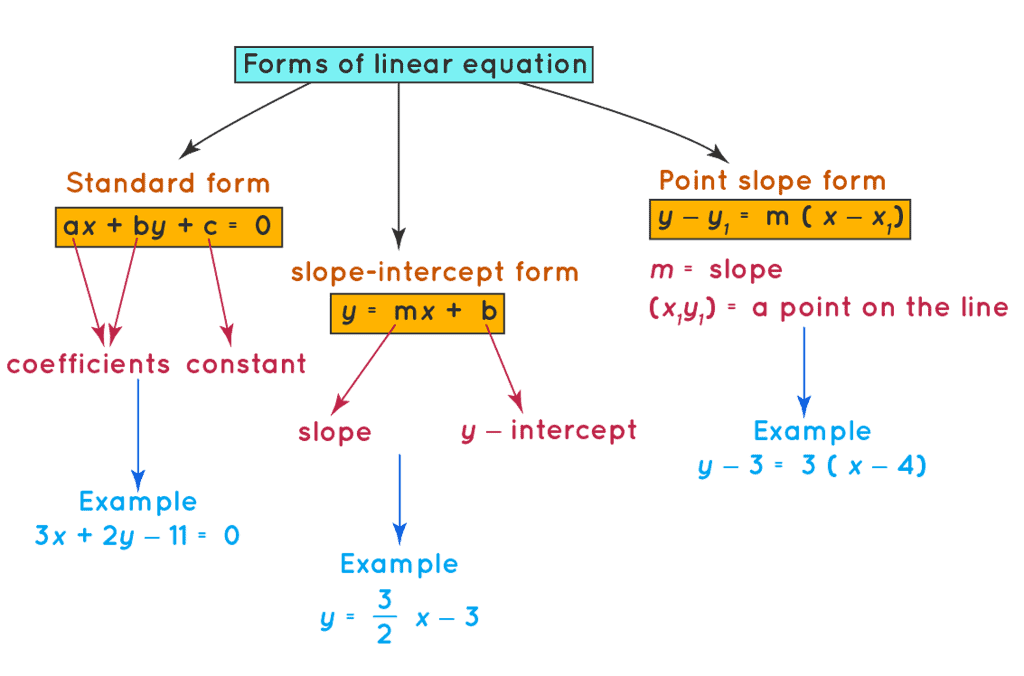Various forms of Linear Equation

The general form of a linear equation in two variables is ax + by + c = 0, where a and b cannot be zero simultaneously.

Let us consider an example of a linear equation in two variables, x + 3y = 7
LHS = x + 3y
If we put x = 1 and y = 2 in the LHS of the equation we get,
LHS = x + 3y = 1 + 3(2) = 7
Here, LHS=RHS
So, we can say that x = 1 and y = 2 is a solution of the equation x + 3y = 7

Let us consider one more value of x and y.
Again putting x = 2 and y = 1 in the LHS of the equation we get,
LHS = x + 3y = 2 + 3(1) = 5
Now, LHS ≠ RHS
Therefore, we say that x = 2 and y = 1 is not a solution of the equation x + 3y = 7.
We know that the graph of a linear equation in two variables is a straight line.
If we say that x = 1 and y = 2 is a solution of the equation x + 3y = 7, then it means that the point (1,2) will lie on the line representing the equation, x + 3y = 7.
Now, we had considered one more case, where the value of x = 2 and y = 1 was not the solution of the equation, x + 3y = 7.
Therefore, we say that the point (2,1) does not lie on the line representing the equation, x + 3y = 7.
So, every solution of the equation is a point on the line representing it.

## Pair of linear equations in two variables

The general form for a pair of linear equations in two variables x and y is
a1x + b1y + c1 = 0
a2x + b2y + c2 = 0

A pair of linear equations in two variables can have one of the following types of solutions:

1. Unique Solution

• When the two equations represent two distinct lines in a coordinate plane, and these lines intersect at a single point, there is a unique solution.
• Mathematically, this means that the lines are not parallel (their slopes are different), and they are not coincident (they do not represent the same line).

2. Infinitely Many Solutions

• If the two linear equations represent the same line in a coordinate plane, they have an infinite number of solutions.
• This occurs when the equations are essentially equivalent, and every point on the common line satisfies both equations.

3. No Solution

• When the two equations represent parallel lines that do not intersect, there is no solution.
• Mathematically, this means that the lines have the same slope (their coefficients are proportional), but they have different y-intercepts.

Question for Chapter Notes: Pair of Linear Equations in two Variables
Try yourself:Which of the following statements is true for a system of linear equations in two variables?

## Graphical Representation of Linear Equation

We know that the geometrical representation of linear equations in two variables is a straight line, but when we have a pair of linear equations then there will be two straight lines, which are considered together.

When there are two lines in a plane, one of the following possibilities may arise.

(i) The two lines will intersect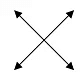(ii) The two lines are parallel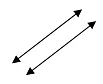(iii) Two lines are coincident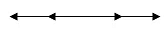## Solving Pair of Linear Equations

Algebraic Methods for solving a Pair of Linear Equations

The graphical method for solving a pair of linear equations is more suitable for integers but for non-integers, it may not be as accurate as we need. So we use other algebraic methods to solve the pair of linear equations.

Some of the algebraic methods which we are going to study now are

• Substitution Method
• Elimination Method

## Substitution  Method

In the substitution method, we find out the value of one variable in terms of the other variable and then we substitute this value in other equations to get an equation in one variable. Now, this equation can be solved easily.
Let us consider some examples to understand the substitution method better.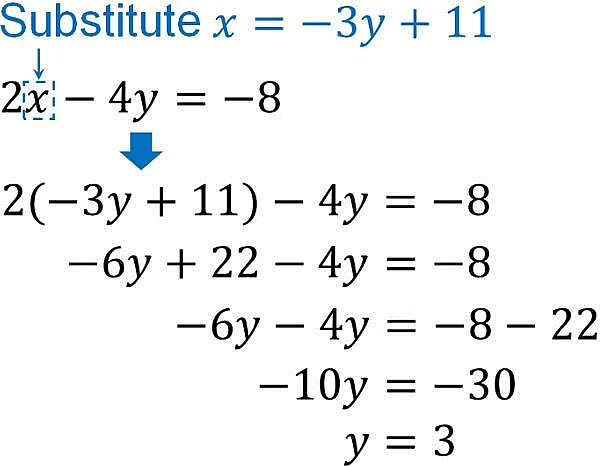Example of Substitution Method

Example 1: Solve the following pair of linear equations by the substitution method.
0. 2x + 0. 3y = 1. 3 and 0. 6x + 0. 5y = 2. 3

Sol:

The given pair of linear equations is
0. 2x + 0. 3y = 1. 3 →Eq 1
0. 6x + 0. 5y = 2. 3 →Eq 2
First, we find the value of variable y in terms of other variables, i.e. x.
From equation (1),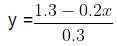Next, we substitute the value of y in equation (2) From equation (2),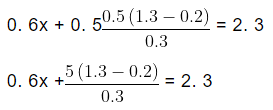1. 8x + (6. 5 − x) = 2. 3 × 3
1. 8x + 6. 5 − x = 6. 9
0. 8x = 6. 9 − 6. 5
0. 8x = 0. 4
x = 0. 5
Putting the value of x in equation 1, we get
0. 2 × 0. 5 + 0. 3y = 1. 3
0. 3y = 1. 3 − 0. 01
0. 3y = 1. 29
y = 4. 3
Therefore, x = 0. 5 and y = 4. 3

Example 2: Solve 2x + 3y = 11 and 2x − 4y = −24 and hence find the value of m for which y = mx + 3.

Sol:

The given pair of linear equations is

2x + 3y = 11 →Eq 1

2x − 4y = −24 →Eq 2

We will first find the value of variable y in terms of variable, x.

From equation (1),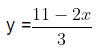Next substituting this value of y in equation (2), we get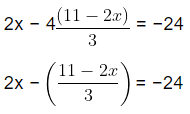6x − (44 − 8x) = −24 × 3
6x + 8x − 44 = −72
14x = −72 + 44
14x = −28
x = −2
Putting the value of x in equation 1, we get
2 × (−2) − 4y = −24
−4 − 4y = −24
−4y = −24 + 4
−4y = −20
y = 5
Therefore, x = −2 and y = 5
Substituting the value of x and y in the equation
y = mx + 3, we get
5 = −2m + 3
m = −1

Example 3: The difference between the two numbers is 75 and on number is four times the other.

Sol:

Let the two numbers be x and y.

x > y
It is given that the difference between x and y is 75.
x − y = 75 → Eq 1
One number is four times the second number.
x = 4y →Eq 2
Substituting the value of x from Eq 2 in Eq 1, we get
4y − y = 75
3y = 75
y = 25
Substituting the value of y in Eq 2, we get

x = 4 × (25)
x = 100
The two numbers are 100 and 25.

Question for Chapter Notes: Pair of Linear Equations in two Variables
Try yourself:Solve the system of equations using the substitution method:

2x + 3y = 9

x - y = 2

## Elimination Method

Elimination Method is another method of eliminating one variable but in this method, one variable out of two variables is eliminated by making the coefficient of that variable equal in both the equations.
Now, we will consider some examples.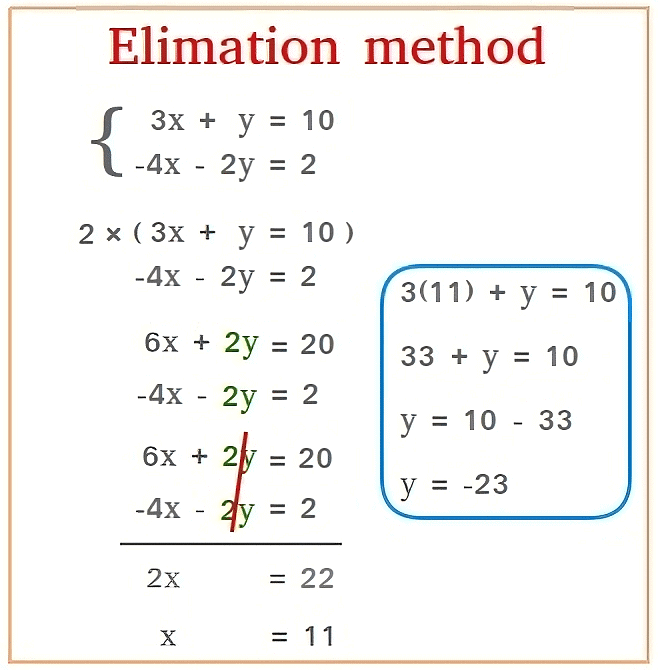Example of Elimination Method

Example 4: Use the elimination method to find all possible solutions of the following pair of linear equations.
ax + by − a + b = 0 and bx − ay − a − b = 0

Sol:

The given pair of linear equations is
ax + by − a + b = 0 → Eq 1
bx − ay − a − b = 0 → Eq 2
Now, we will multiply Eq 1 by b and Eq 2 by a to make the coefficient of x equal
bax + b2y − ab + b2 = 0 → Eq 3
abx − a2y − a2 − ab = 0 → Eq 4
Subtract Eq 3 from Eq 4 to eliminate x, as the coefficients of x are the same
bax + b2y − ab + b2 − (abx − a2y − a2 − ab) = 0
(ba − ab)x + (b2 + a2)y − ab + b2 + a+ ab = 0
(b2 + a2)y = −(b2 + a2)
y =−(b2 + a2)b2 + a2
y = −1
Putting y = 1 in Eq 2 we get,
bx − a(−1) − a − b = 0
bx + a − a − b = 0
bx − b = 0
x = 1
Therefore, x = 1, and y = −1 which is the required unique solution of the given pair of linear equations.

Example 5: The sum of the digits of a two-digit number is 6. Also, seventeen times this number is five times the number obtained by reversing the order of the digits. Find the number.

Sol:

Let the digit at the unit place be x and the digit at tens place be y.
The Original two-digit number is 10y + x
The sum of the digits of the original number = 6
y + x = 6
x + y − 6 = 0 → Eq 1
On reversing the order of the two-digit number, we get y at units place and x at the tens place.
∴ The new two-digit number= 10x + y
Seventeen times of the original number is equal to five the reversed number
17(10y + x) = 5(10x + y)
170y + 17x = 50x + 5y
170y − 5y = 50x − 17x
165y = 33x
x = 5y
x − 5y = 0 → Eq 2
On subtracting Eq 2 from Eq 1, we get
x + y − 6 − (x − 5y) = 0

x + y − 6 − x + 5y = 0
6y = 6
y = 1
Substituting y = 1 in Eq 1
x + 1 − 6 = 0
x = 5
Therefore, the required two-digit number = 10y + x = 10 × 1 + 5 = 15

Example 6: A fraction becomes 5/7 , if 2 is subtracted to both the numerator and the denominator. If 3 is subtracted to both the numerator and denominator, it becomes 2/3. Find the fraction.

Sol:

Let the fraction be x/y
Subtracting 2 to both the numerator and denominator, we get new fraction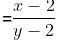It is given that the new fraction obtained =5/7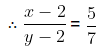7(x − 2) = 5(y − 2)

7x − 14 = 5y − 10

7x − 5y = 14 − 10

7x − 5y = 4

7x − 5y − 4 = 0 → Eq 1

Next, we subtract 3 to both the numerator and denominator New fraction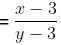It is given that the new fraction obtained = 2/3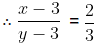3(x − 3) = 2(y − 3)
3x − 9 = 2y − 6
3x − 2y = 3
3x − 2y − 3 = 0 → Eq 2
7x − 5y − 4 = 0 → Eq 1
Multiplying Eq 1 by 3 and Eq 2 by 7 to make the coefficient of x equal, we get
21x − 15y − 12 = 0 → Eq 3
21x − 14y − 21 = 0 → Eq 4
Now, on subtracting, we get
21x − 15y − 12 − (21x − 14y − 21) = 0
21x − 15y − 12 − 21x + 14y + 21 = 0
−y + 9 = 0
y − 9 = 0
y = 9
Putting the value of y in Eq 1, we get
7x − 5(9) − 4 = 0
7x − 45 − 4 = 0
7x = 49
x = 7

Therefore the required fraction is 7/9 .

Example 7: The taxi charges in a city consist of a fixed charge together with the charge for the distance covered. For a distance of 10km, the charge paid is Rs. 110 and for a journey of 15 km the charge paid is Rs.160. What are the fixed charges and the charge per kilometer? How much does a person have to pay for traveling a distance of 25 km?

Sol:

Let fixed charge be Rs. x and charge per kilometer be Rs. y For 10 km distance, Fixed charge + charge per kilometer = Rs. 110
x + 10y = 110
x + 10y − 110 = 0 → Eq 1
For 15 km distance,
Fixed charge + charge per kilometer = Rs. 16
x + 15y = 160
x + 15y − 160 = 0 → Eq 2
Now subtracting Eq 2 from Eq 1 we get,
x + 15y − 160 − (x + 10y − 110) = 0
x + 15y − 160 − x − 10y + 110 = 0
15y − 10y − 50 = 0
5y − 50 = 0
y = 10
Substituting the value of y in Eq 1 we get,
x + 10y − 110 = 0
x + 10(10) − 110 = 0
x + 100 − 110 = 0
x = 10
Therefore, the fixed charge is Rs. 5 and the charge per kilometer is Rs. 10.
For 25 km distance,
Fixed charge + charge per kilometer = Rs. x + 25y
= Rs 10 + 25 (10)
= Rs 260

Question for Chapter Notes: Pair of Linear Equations in two Variables
Try yourself:Solve the system of equations using the elimination method:

2x + 3y = 10

3x - 2y = 2

## Cross Elimination  Method (Deleted from NCERT)

Cross Multiplication Method is another useful method of solving a linear pair of linear equations.
To solve a pair of linear equations by cross multiplication method following steps are followed,
We know the standard form of pair of linear equations is
a1x + b1y + c1 = 0 → Eq 1
a2x + b2y + c2 = 0 → Eq 2
Now we multiply Eq 1 by b2 and Eq 2 by b1 to get,
b2a1x + b2b1y + b2c1 = 0 → Eq 3
b1a2x + b1b2y + b1c2 = 0 → Eq 4
Next, we subtract Eq 4 from Eq 3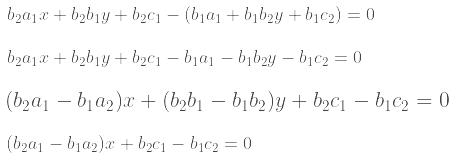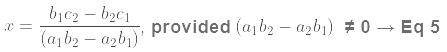Now we substitute the value of x in Eq 1to get,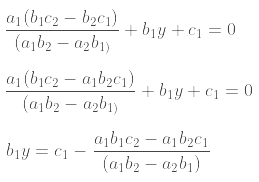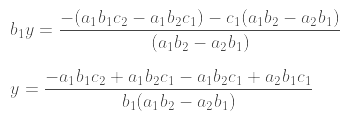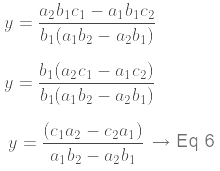The above solution is commonly written as,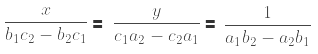Now two different cases may arise,
Case 1: (a1b2 − a2b1 ) ≠ 0.
a1b2 ≠ a2b1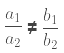In this case, the pair of linear equations has a unique solution.
Case 2: (a1b2 − a2b1) = 0
a1b2 = a2b1,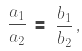then there are two conditions
(i) If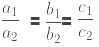then the pair of linear equations has infinitely many solutions.
(ii) If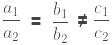then the pair of linear equations has no solutions.
The diagram given below is useful in memorizing the method of cross-multiplication.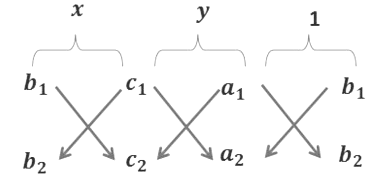The arrow between the numbers indicates that they are to be multiplied and the second product is to be subtracted from the first.
Therefore we get,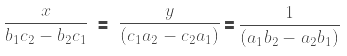Example 8: Solve the following pair of linear equations by Cross- Multiplication Method.
8x + 5y = 9, 3x + 2y = 4

Sol:

The given pair of linear equation is
8x + 5y = 9
8x + 5y − 9 = 0 → Eq 1
3x + 2y = 4
3x + 2y − 4 = 0 → Eq 2

We know the standard form of pair of linear equations is
a1x + b1y + c1 = 0 → Eq 3
a2x + b2y + c2 = 0 → Eq 4
Next we compare the given pair of linear equations with the standard form of linear equation.
So, we compare Eq 1 with Eq 3 and Eq 2 with Eq 4 respectively to get,
a1 = 8, a2 = 3, b1 = 5, b2 = 2, c1 = −9, c2 = −4
To solve the equations by cross multiplication method, we draw the diagram given below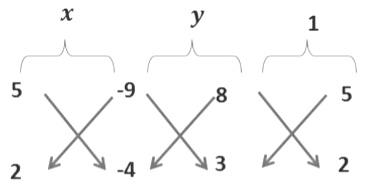Therefore we get,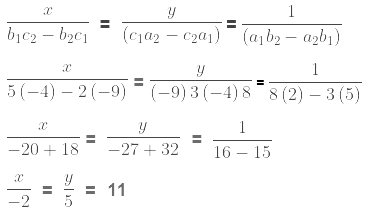Therefore, x = −2 and y = 5

Example 9: For which values of a and b does the following pair of linear equations have an infinite number of solutions.

2x + 3y = 7 and (a − b)x + (a + b)y = 3a + b − 2

Sol:

The given pair of linear equation is
2x + 3y = 7
2x + 3y − 7 = 0 → Eq 1
(a − b)x + (a + b)y = 3a + b − 2
(a − b)x + (a + b)y − 3a − b + 2 = 0 → Eq 2
Next, we compare the given pair of linear equations with the standard form of linear equation,
a1x + b1y + c1 = 0 → Eq 3
a2x + b2y + c2 = 0 → Eq 4
On comparing Eq 1with Eq 3 and Eq 2 with Eq 4, we get
a1 = 2, a2 = (a − b), b1 = 3, b2 = (a + b), c1 = −7, c2 = −3a − b + 2
For an infinite number of solutions we have
If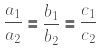then the pair of linear equations has infinitely many solutions.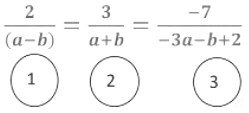Taking 1 and 2 terms we get,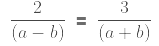2(a + b) = 3(a − b)
2a + 2b = 3a − 3b
2a − 3a = −3b − 2b
−a = −5b
a = 5b → Eq 3
Taking 2 and 3 we get,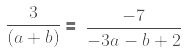3(−3a − b + 2) = −7(a + b)
−9a − 3b + 6 = −7a − 7b
−9a + 7a − 3b + 7b = −6−2a + 4b = −6
Dividing the above equation by 2 we get,
−a + 2b = −3 → Eq 4
Putting a = 5b in the above equation we get,
−5b + 2b = −3
−3b = −3
b = 1
Putting the value of b in → Eq 3 we get
a = 5(1) = 5
Therefore, a = 5, and b = 1

Example 10: The ratio of incomes of two persons is 9:7 and the ratio of their expenditures is 4:3. If each of them saves Rs 200 per month, find their monthly incomes.

Sol:

Let the monthly income of the two persons be 9x and 7x respectively.
Let their monthly expenditure be 4y and 3y respectively.
Monthly Savings = Monthly Income – Monthly expenditure
Monthly Savings of the first person=9x − 4y
Monthly Savings of the second person= 7x − 3y
9x − 4y = 200
9x − 4y − 200 = 0 → Eq 1
7x − 3y = 200
7x − 3y − 200 = 0 → Eq 2
The standard form of pair of linear equations is
a1x + b1y + c1 = 0 → Eq 3
a2x + b2y + c2 = 0 → Eq 4
Next, we compare the given pair of linear equations with the standard form of linear equations.
So, we compare Eq 1 with Eq 3 and Eq 2 with Eq 4 respectively to get,

a1 = 9, a2 = 7, b1 = −4, b2 = −3, c1 = −200, c2 = −200 .
By using cross multiplication method we get,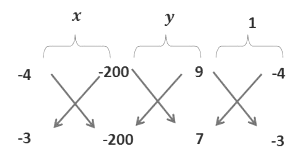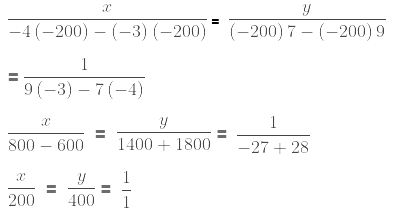x = 200
y = 400
Monthly income of first person = 9x = 9 × 200 = Rs.1800
Monthly income of second person = 7x = 7 × 200 = Rs. 1400

## Solving Pair of Linear Equations - Graphical Method (Deleted from NCERT)

Graphical Method of Solution of a Pair of Linear Equations

We know that a pair of linear equations is represented graphically by two straight lines and these lines may be parallel, may intersect or may coincide.

Now, we will consider certain cases here,

• If the two lines are intersecting each other at one point only, then we say that there is one and only one solution, that is, a unique solution exists for this pair of linear equations in two variables. Such a pair of linear equations is called a consistent pair of Linear equations.
• If the two lines are coincident then we say that the pair of linear equations has infinitely many solutions. Such a pair of linear equations is called an inconsistent pair of Linear equations.
• If the two lines are parallel to each other, that is, they do not meet at all, and then we say that the two linear equations have no common solution. Such a pair of linear equations is called a dependent pair of Linear equations.

If the lines represented by the equation,

a1x + b1y + c1 = 0
a2x + b2y + c2 = 0 are,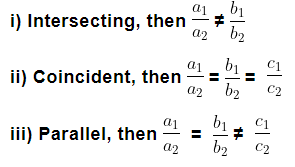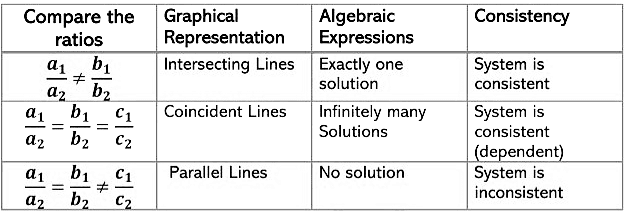Let us consider some examples here, to understand better the above relations.

Example 13: Solve graphically the pair of linear equations 3x − 4y + 3 = 0 and 3x + 4y − 21 = 0. Find the coordinate of the vertices of the triangular region formed by these lines and X-axis. Also, calculate the area of this triangle.

Sol:

Firstly we will find two solutions for each equation.

(i) Table for 3x − 4y + 3 = 0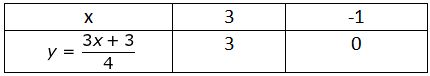Let the points be A (3, 3) and B (-1,0)

(ii) Table for 3x + 4y − 21 = 0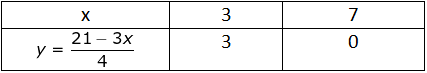Let the points be C (3, 3) and D (7,0)

Now we will plot these points on a graph paper.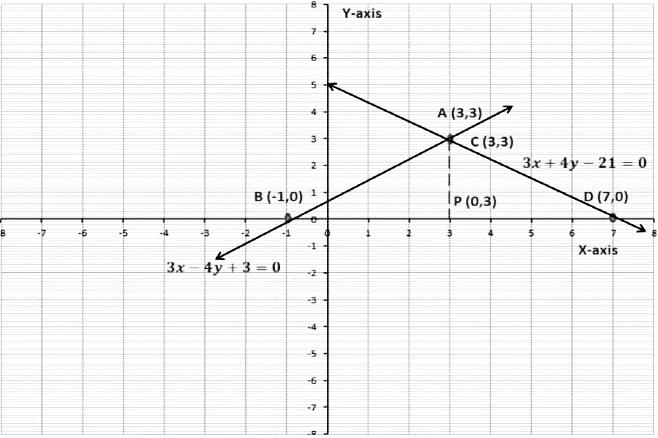We will join points A and B, A and D respectively.

(Point A and Point C have the same coordinate)

When we join these points we will get a triangle ∆ ABD.

The coordinates of the vertices of ∆ ABD are,

A (3,3), B (-1,0) and D (7,0)

Next, we have to find the area ∆ ABD.

We know,

Area of a triangle =  1/2 × Base × Height

In ∆ ABD,

Base = 8 units

Height = 3 units

Area of ∆ ABD = 1/2 ×BD×AP

=12× 8 ×3 = 12 sq. units

Example 14: Solve the following system of linear equations graphically:
2x − y − 4 = 0
x + y + 1 = 0

Sol:

The pair of linear equations is,

2x − y − 4 = 0

x + y + 1 = 0

First, we will find two solutions of each equation.

(i) Table for 2x − y − 4 = 0

Let the points be A(0,-4) and B(2,0)

(ii) Table for x + y + 1 = 0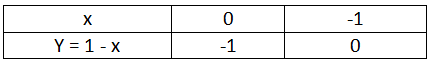Let the points be C (0,-1) and D (-1,0)

Now we plot these points on a graph paper.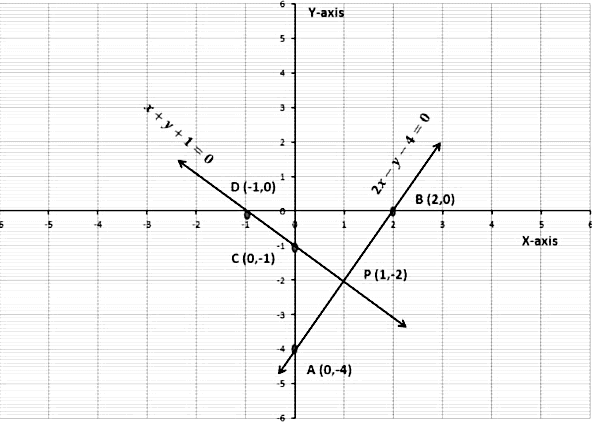We have joined points A and B, C and D respectively.
We see that the two lines are intersecting at point P (1, -2).
So the solution of the given pair of equations is x = 1 and y =−2.

Example 15: Form a pair of linear equations in two variables using the following information and solve it graphically. Five years ago, Mayank was twice as old as Rajat. Ten years later, Mayank’s age will be ten years more than Rajat’s age. Find their present ages.

Sol:

Let Mayank’s and Rajat’s present age be x years and y years respectively.

5 years ago,

Mayank’s age was (x − 5) years

Rajat’s age was (y − 5) years

Now it is given that 5 years ago Mayank was twice as old as Rajat.
∴ (x − 5) = 2(y − 5)
x − 5 = 2y − 10
x − 2y = −10 + 5
x − 2y = −5 → Eq.1
10 years later,
Mayank’s age will be (x + 10) years
Rajat’s age was (y + 10) years
It is given that 10 years later, Mayank’s age will be ten years more than Rajat’s age.

∴ (x + 10) = (y + 10) + 10
x + 10 = y + 20
x − y = 10 → Eq.2
We get a pair of linear equations,
x − 2y + 5 = 0
x − y − 10 = 0
Now we draw the graph of Equations 1 and 2.
We will find two solutions of the two equations first
Table for x − 2y + 5 = 0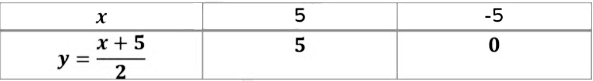Let the points be A (5, 5) and B(-5,0)

Table for x − y − 10 = 0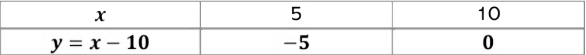Let the points be C (5,-5) and D (10,0)

Now we plot these points on a graph paper.

Join points A and B to get, line AB

Join points C and D to get, line CD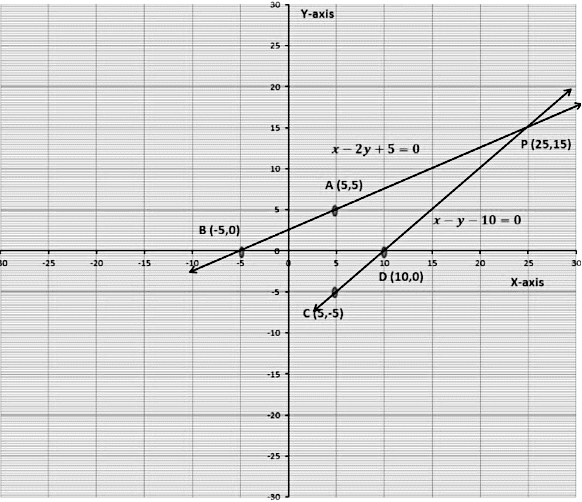We see that the lines AB and CD are intersecting at point P. The coordinate of points of P is (25, 15), that is, x = 25 years and y = 15 years

Mayank’s age = 25 years

Rajat’s age = 15 years

Example 16: Check whether the given pair of linear equations is consistent or inconsistent. x + 2y = 4 and 3x + 6y = 12

Sol:

The pair of linear equations is

x + 2y = 4

3x + 6y = 12

The standard form of pair of linear equations is

a1x + b1y + c1 = 0

a2x + b2y + c2 = 0

On comparing the linear equations with the standard form of equations we get,

a1 = 1, b1 = 2 and c1 = 4

a2 = 3, b2 = 6 and c2 = 12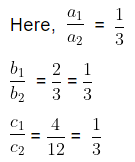We see that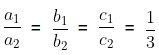Therefore, the given pair of linear equations is consistent.

Example 17: Find the value of ‘k’ for which the system of equations kx − 5y = 2 and 6x + 2y = 7 has no solution.

Sol:

The given pair of linear equations is,
kx − 5y = 2
6x + 2y = 7
We know the standard form of pair of linear equations is
a1x + b1y + c1 = 0
a2x + b2y + c2 = 0
On comparing the linear equations with the standard form of equations we get,

a1 = k, b1 = −5 and c1 = 2
a2 = 6, b2 = 2 and c2 = 7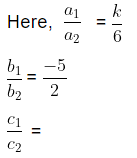We know that a pair of linear equations has no common solution when,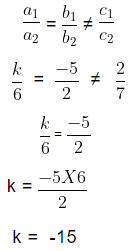## Equations Reducible to Linear Equations in Two Variables (Deleted from NCERT)

Equations Reducible to a pair of Linear Equations in Two Variables
Some equations are not linear but can be reduced to a pair of linear equations by making suitable substitutions. Let us consider some examples.

Example 18: Solve for x and y.
x + y = 18xy and x + 2y = 21xy

Sol:

The given pair of linear equations is
x + y = 18xy
x + 2y = 21xy
On dividing Eq 1 and Eq 2 by xy we get,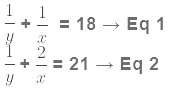These equations are not in the formax + by + c = 0. If we substitute 1/y = p and 1/x = q in Eq 1 and Eq 2 we get,
p + q = 18 → Eq 3
p + 2q = 21 → Eq 4
On subtracting Eq 3 from Eq 4 we get,
p + 2q − (p + q) = 21 − 18
p + 2q − p − q = 21 − 18
p − p + 2q − q = 3
q = 3
q = 3
Putting q = 3 in Eq 3 we get,
p + (3) = 18
p = 18 − 3
p = 15
Now, 1/y = p
1/y = 15 ⇒ y = 1/15
1/x = q
1/x = 3 ⇒ x = 1/3
Therefore, x = 1/3 and y = 1/15 is the required solution.

Example 19: If 2 times the area of a smaller square is subtracted from the area of a larger square, the result is 16 m2. The sum of the area of the two squares is 208 m2. Determine the side of the two squares.

Sol:

Let the area of the smaller square be x2  and the area of the larger square be y2.

On subtracting the area of the smaller square from the area of the larger square we get,

y2 − 2x2 = 16 m2 → Eq 1

On adding the area of the two squares we get,y2 + x2 = 208 m2 → Eq 2.

To convert Eq 1 and Eq 2 to linear equations we assume that,

x2 = p and y2 = q.

Eq 1 becomes,
q − 2p = 16

q − 2p − 16 = 0 → Eq 3

Eq 2 becomes,
q + p = 208

q + p − 208 = 0 → Eq 4

Now, subtracting Eq 3 from Eq 4, we get
q + p − 208 − (q − 2p − 16) = 0
q + p − 208 − q + 2p + 16 = 0
3p − 192 = 0
p = 64
Putting the value of p in Eq 4 we get,
q + 64 − 208 = 0
q − 144 = 0
q = 144

Now we know that x2 = p and y2 = q

x2 = p ⇒ x2 = 64
x = 8y2 = q ⇒ y2 = 144
y = 12
Therefore, the side of the smaller square is 8 m and the side of the larger square is 12 m.

Example 20: Ritu can row downstream 20 km in 2 h and upstream 4 km in 2 h. Find her speed of rowing in still water and the speed of the current.

Sol:

Let the speed of rowing in still water be x km\hr and the speed of current be y km\hr.
Downstream speed = (x + y) km\hr
Upstream speed = (x − y) km\hr
When Ritu is rowing downstream then,
Time taken = 2 h
Distance covered = 20 km
Speed= (x + y) km\hr
We know, Time =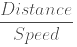2 =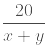2 (x + y) = 20
x + y = 10 .................................................Eq 1
When Ritu is rowing upstream then,
Time taken = 2 h
Distance covered = 4 km
Speed= (x − y) km\hr
We know, Time =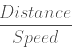2 =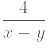2 (x − y) = 4
x − y = 2 ....................................................Eq 2
Subtracting Eq 1 from Eq 2 we get,
x − y − (x + y) = 2 − 10
x − y − x − y = −8
x − x − y − y = −8
−2y = −8
y = 4
Putting y = 4 in Eq 2 we get,
x − 4 = 2
x = 6
Hence, the speed of rowing in still water is 6 km\hr and the speed of the current is 4 km\hr.

The document Pair of Linear Equations in two Variables Chapter Notes | Mathematics (Maths) Class 10 is a part of the Class 10 Course Mathematics (Maths) Class 10.
All you need of Class 10 at this link: Class 10

## Mathematics (Maths) Class 10

115 videos|478 docs|129 tests

## FAQs on Pair of Linear Equations in two Variables Chapter Notes - Mathematics (Maths) Class 10

 1. What is the definition of linear equations?Ans. Linear equations are algebraic equations that involve two variables and can be written in the form ax + by = c, where a, b, and c are constants.
 2. How are linear equations represented graphically?Ans. Linear equations can be represented graphically by plotting the points on a coordinate plane and connecting them with a straight line. The line represents all the solutions of the equation.
 3. What is the substitution method for solving a pair of linear equations?Ans. The substitution method involves solving one equation for one variable and then substituting that expression into the other equation. This allows us to solve for the remaining variable and find the solution to the pair of equations.
 4. How does the elimination method work for solving a pair of linear equations?Ans. The elimination method involves adding or subtracting the two equations in such a way that one variable gets eliminated. This results in a new equation with only one variable, which can be solved to find its value. Substituting this value into either of the original equations gives the value of the other variable.
 5. What is the graphical method for solving a pair of linear equations?Ans. The graphical method involves plotting the graphs of the two equations on a coordinate plane and finding the point of intersection. This point represents the solution to the pair of equations.

## Mathematics (Maths) Class 10

115 videos|478 docs|129 testsExplore Courses for Class 10 examSignup to see your scores go up within 7 days! Learn & Practice with 1000+ FREE Notes, Videos & Tests.
10M+ students study on EduRev
Track your progress, build streaks, highlight & save important lessons and more!
Related Searches

,

,

,

,

,

,

,

,

,

,

,

,

,

,

,

,

,

,

,

,

,

;